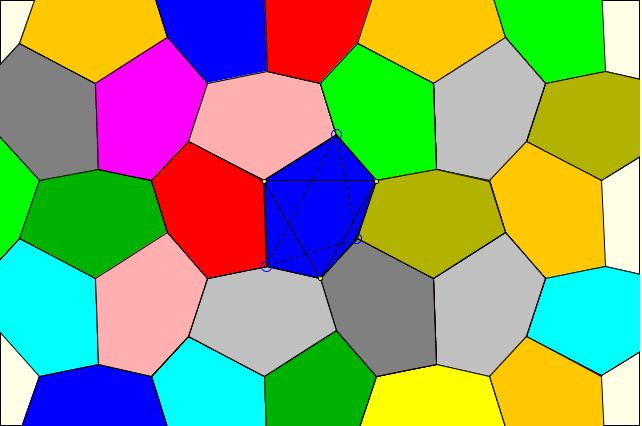# Escher's Theorem

The now famous Dutch graphic artist M. C. Escher was fond of and made a frequent use of plane tessellations, sometimes by the copies of the same figure. One particular tessellation with a special kind of hexagon has been mentioned in his notebooks and now is part of Escher's theorem (after J. F. Rigby.) Here that theorem with a slight change of notations.

### Theorem

1. Let A'B'C' be an equilateral triangle and B any point. Let C be the point such that A'B = A'C and ∠CA'B = 120°. Let A be such that B'A = B'C and ∠CB'A = 120°. Then C'A = C'B and ∠AC'B = 120°.
2. Congruent copies of hexagon AC'BA'CB' can be used to tessellate the plane.
3. The line AA', BB', CC' are concurrent.

As Rigby observed, the theorem was not stated accurately because no assumption had been made as to the orientation of the three 120° angles used in the construction nor the orientation of ΔA'B'C'. For the theorem to work those orientations must be the same. (They are the same in the applet below.) In all likelihood, Escher had this in mind but did not mention in his Notes.

### This applet requires Sun's Java VM 2 which your browser may perceive as a popup. Which it is not. If you want to see the applet work, visit Sun's website at https://www.java.com/en/download/index.jsp, download and install Java VM and enjoy the applet.What if applet does not run?

To prove the first part, consider Napoleon triangles ABC'', AB''C abd A''BC. By Napoleon's theorem, their centers form an equilateral triangle. A' and B' are two of the vertices of that equilateral triangle and since ΔA'B'C' is equilateral, C' is the third vertex.

The applet serves to illustrate the second part of the claim. The tessellation can be obtained from the Napoleon's tessellation we discussed elsewhere bu joining vertices of the equlateral triangles to their centers. (To see that in the applet, check both Show tessellation and Hint boxes.)

The third part is the content of Kiepert's theorem.

### References

1. J. F. Rigby, Napoleon, Escher, and Tessellations, Mathematics Magazine, Vol. 64, No. 4, (Oct., 1991), pp. 242-246### Napoleon's Theorem### Plane Tessellations

• Dancing Squares or a Hinged Plane Tessellation
• Dancing Rectangles Model Auxetic Behavior
• A Hinged Realization of a Plane Tessellation
• A Semi-regular Tessellation on Hinges A
• A Semi-regular Tessellation on Hinges B
• A Semi-regular Tessellation on Hinges C
• Napoleon Theorem by Plane Tessellation
• Parallelogram Law: A Tessellation
• Simple Quadrilaterals Tessellate the Plane
• Pythagorean Theorem By Plane Tessellation
• Pythagorean Theorem a la Friedrichs
• Pythagorean Theorem By Hexagonal Tessellation
• Hinged Greek Cross Tessellation
• Pythagorean Theorem: A Variant of Proof by Tessellation
•